### GATE 2000 ECE Video Solutions on Analog Circuits (Analog Electronics)

1. In the differential amplifier of the figure, if the source resistance of the current source IEE is infinite, then the common mode gain isa. Zero
b. Infinite
c. Indeterminate
d. (Vin1 + Vin2) / 2VT
Solution: https://www.youtube.com/watch?v=goC-ZRrr_Yw

2. In the circuit shown in figure, the output voltage Vo isa. – 1 volts
b. 2 volts
c. + 1 volts
d. + 15 volts
Solution: https://www.youtube.com/watch?v=cWDErAgRgyE

3. Introducing a resistor in the emitter of a CE amplifier stabilizes the dc operating point against variations in
a. Only the temperature
b. Only the β of the transistor
c. Both temperature and β
d. None of the above
Solution: https://www.youtube.com/watch?v=Hj4RgZDwBwk

4. The current gain of a bipolar transistor drops at high frequencies because of
a. Transistor capacitances
b. High current effects in the base
c. Parasitic inductance effects
d. The Early Effect
Solution: https://www.youtube.com/watch?v=mZE9xTPfxjs

5. If the OP-AMP in the figure is ideal, then the output voltage Vo is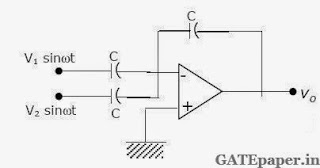a. Zero
b. (V1 – V2) sinωt
c. – (V1 + V2) sinωt
d. (V1 + V2) sinωt
Solution: https://www.youtube.com/watch?v=WCAhUYmqiWA

6. The configuration of the figure is aa. Precision rectifier
b. Hartley oscillator
c. Butterworth high pass filter
d. Wien-bridge oscillator
Solution: https://www.youtube.com/watch?v=PxZImxr0h6Q

7. Assume that the OP-AMP of the figure is ideal. If Vi is a triangular wave, then Vo will bea. Square wave
b. Triangular wave
c. Parabolic wave
d. Sine wave
Solution: https://www.youtube.com/watch?v=ZvsEb1Tpxaw

8. The most commonly used amplifier in Sample and Hold circuits is
a. Unity gain inverting amplifier
b. Unity gain non-inverting amplifier
c. An inverting amplifier with a gain of 10
d. An inverting amplifier with a gain of 100
Solution: https://www.youtube.com/watch?v=JFfOcIr8x7U

9. If the OP-AMP in the figure has an input offset voltage of 5 mV and an open loop voltage gain of 10,000. Then the output Vo will be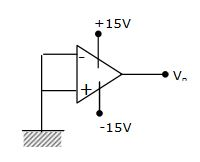a. 0 volts
b. 5 mV
c. + 15 volts or – 15 volts
d. + 50 volts or – 50 volts
Solution: https://www.youtube.com/watch?v=EXEzhngomhY

10. a. For the circuit shown, plot Vo under steady state conditions, with and without capacitor C. Assume that the diode is ideal.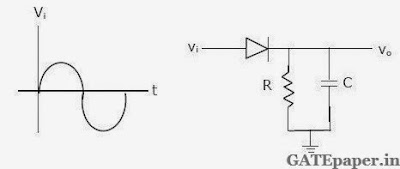Solution: https://www.youtube.com/watch?v=GJXs26_62eE

b. Design a circuit using two ideal diodes, one resistor and two voltage sources that would convert the input voltage to the output voltage as shown in figure. The resistor value need not be specified.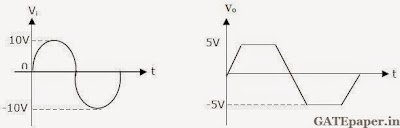Solution: https://www.youtube.com/watch?v=gXD0ms6S8iY

11. For the amplifier circuit shown, IC = 1.3 mA, RC = 2 kΩ, RE = 500 Ω, VT = 26 mV, β = 100, VCC = 15 volts, VS = 0.01 sin(ωt) volts and Cb = Ce = 10 µF.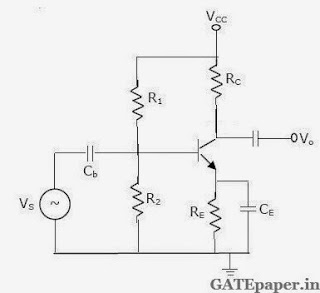a. What is the small signal voltage gain, Vo/VS
b. What is the approximate voltage gain if Ce is removed?
c. What will be the output Vo, if Cb is short circuited?
Solution: https://www.youtube.com/watch?v=x75WPMXrTOg

12. For a feedback amplifier, the open loop transfer function has three poles at 100 k rad/sec, 1 M rad/sec and 10 M rad/sec. The low frequency open loop gain is 1000 and the feedback factor (β) is 1. Use Bode plots to determine the phase margin of the amplifier. Is the amplifier stable?

Solution: Amplifier is Unstable.

13. Below figure shown is a common base amplifier.a. Write expressions for the time constants associated with the capacitances Cb and Cs.
b. What is the approximate lower cutoff frequency of the amplifier?
Solution: https://www.youtube.com/watch?v=wTtlORiy598

Name

Email *

Message *